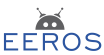#Real-Time Robotics Framework

### Sidebar

tools:matrix:start

This is an old revision of the document!

# Vectors and Matrices

The blocks in the control system are connected among each other with the aid of signals. As described in Signals they carry a timestamp and a value. The value could be a simple type such as an integer or float. Quite often it will consist of a vector or a matrix. A three dimensional vector of type double can be declared and initialized as follows:

Vector<3, double> v;
v << 1.5, -2, 0;

The declaration could be simplified to

Vector3<> v;
v << 1.5, -2, 0;

as Vector3 is a predefined type with three dimensions and the default element type is double. A matrix of 3 times 3 with element type int could be defined as

Matrix<3, 3, int> m;
m << 1, 4, 7,
2, 5, 8,
3, 6, 9;

The first three numbers will be filled into the first colon. While the internal representation is simply a one dimensional vector, the matrix could be visualized as

col0col1col2
row0123
row1456
row2789

## Accessing Elements

You can access rows, columns or single elements of matrices with the following methods:

m.get(0,0);  // returns element, 1
m.getRow(1);  // returns row, [2,5,8]
m.getCol(0);  // returns column, [1,2,3]'
m.getSubMatrix<2,2>(0,1);   // returns matrix [[4,5][7,8]]

Single elements, rows or columns can be written with the methods set(), setRow(), or setCol().

The operators () and [] work as well as can be seen by the following example

m(3);   // returns element or sets element with index 3
m;   // returns element or sets element with index 3
m(2,1);   // returns element or sets element with index 7
m[2,1];   // does not work, as the [] operator cannot be defined for two parameters

## Logging Matrices

A matrix can be logged simply by writing

log.info() << m;

With the 3 x 3 matrix from above we would get

2021-09-16 17:36:53:929    E:  [ [1 4 7]' [2 5 8]' [3 6 9]' ]

The matrix is plotted colon per colon.